Next: 4.4.2 Numerical Backward Transform Up: 4.4 Numerical Implementation Previous: 4.4 Numerical Implementation

## 4.4.1 Alias Free'' Forward Transform

The basic idea is to decompose the mask transmission function t(x, y) into elementary geometrical patterns. Triangles are best suited for this purpose because any function t(x, y) consisting of polygonal-shaped, piecewise constant transmission areas can be triangulated and an analytical expression for the Fourier coefficients of a triangular-shaped area can easily be derived (cf. Appendix B.1). As a typical photomask often consists of rectangular areas we consider them as the second type of elementary patterns. Formulae for the Fourier coefficients of rectangles are presented in Appendix B.2.

Let us now find a mathematical description for the proposed algorithm. The area of a general elementary pattern is described by its indicator function PAT(x, y) defined as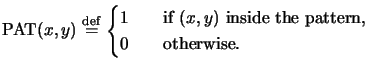(4.91)

In our situation the elementary patterns are either triangles or rectangles. In principle any other pattern type can be used as long as analytical expressions for its Fourier coefficients can be derived.o The decomposition of the mask transfer function formally writes as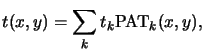(4.92)

whereby tk is the transmission of the respective elementary pattern. In the case of binary masks all transmissions tk equal unity, for phase-shifting masks tk is a complex number with module less or equal one, i.e.,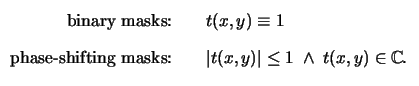(4.93)

Due to the linearity of the Fourier transform the coefficients Tnm of the mask transmission function t(x, y) can be computed as follows:p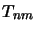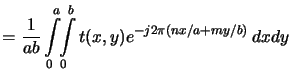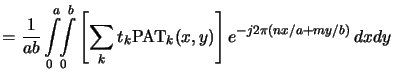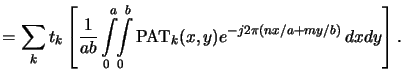(4.94)

The term in square brackets in the last row, i.e.,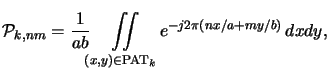(4.95)

can be calculated analytically for the elementary patterns (cf. Appendix B) and the Fourier coefficients Tnm follow to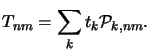(4.96)

In Figure 4.10 the proposed procedure is demonstrated for a simple triangulated mask pattern . As mentioned before, the algorithm has the big advantage that the Fourier coefficients Tnm are calculated exactly, i.e., no sampling of the mask transfer coefficients is involved. A pertubation analysis of the analytical formulae shows that the formulae are numerically robust (cf. Appendix B.3). In addition to accuracy speed is the second important benefit. The calculation is faster when compared to a numerical FFT transform, because the formulae for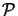k, nm can easily be evaluated (cf. Appendix B). This second point is especially important for a sparse layout as the mask can be decomposed into a relatively small number of geometrical patterns.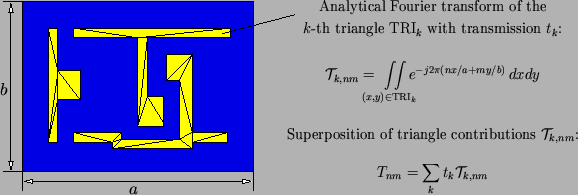#### Footnotes

... derived.o
The Fourier coefficients of polygonal areas can always be computed analytically; circular or elliptic areas can also be treated analytically.
... follows:p
We assume here that the mask transmission function is already scaled with the magnification M of the projection printing system.Next: 4.4.2 Numerical Backward Transform Up: 4.4 Numerical Implementation Previous: 4.4 Numerical Implementation
Heinrich Kirchauer, Institute for Microelectronics, TU Vienna
1998-04-17